### IMO Shortlist 2006 problem G7

Kvaliteta:
Avg: 0,0
Težina:
Avg: 9,0
In a triangle$ABC$, let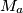$M_{a}$,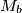$M_{b}$,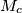$M_{c}$ be the midpoints of the sides$BC$,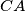$CA$,$AB$, respectively, and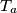$T_{a}$,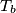$T_{b}$,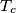$T_{c}$ be the midpoints of the arcs$BC$,$CA$,$AB$ of the circumcircle of$ABC$, not containing the vertices$A$,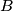$B$,$C$, respectively. For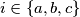$i \in \left\{a, b, c\right\}$, let$w_{i}$ be the circle with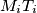$M_{i}T_{i}$ as diameter. Let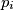$p_{i}$ be the common external common tangent to the circles$w_{j}$ and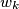$w_{k}$ (for all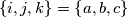$\left\{i, j, k\right\}= \left\{a, b, c\right\}$) such that$w_{i}$ lies on the opposite side of$p_{i}$ than$w_{j}$ and$w_{k}$ do.
Prove that the lines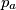$p_{a}$,$p_{b}$,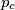$p_{c}$ form a triangle similar to$ABC$ and find the ratio of similitude.
Izvor: Međunarodna matematička olimpijada, shortlist 2006